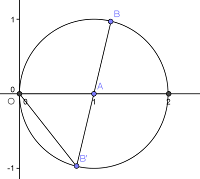# 对 ∏sin(kπ/n) 的小小探索

(z – z1) (z – z12) … (z – z1n-1) = 1 + z + z2 + … + zn-1

= |(1 – z1) (1 – z12) … (1 – z1n-1)|

= |(1 – z1)| |(1 – z12)| … |(1 – z1n-1)|OB’ = OAAB, 表示1 – z1k

∴ 左边 = 2n-1 sin(π/n) sin(2π/n) … sin((n-1)π/n) = 右边 = n

∴ sin(π/n) sin(2π/n) … sin((n-1)π/n) = n / 2n-1.

n / 2n-1 即为(*)式的值

= (sin2° sin4° sin6° … sin86° sin88°) / (sin4° sin8° sin16° … sin88°)

= √(90/2^89) / (45/2^44)

= 1 / 222

### 4 Replies to “对 ∏sin(kπ/n) 的小小探索”

1.Yang Qiuyu says:

看不动题目… 不应该是短频快吗

1.louyiwen says:

题目不是简洁明了吗……
哈哈，短平快这个我们度娘过了http://baike.baidu.com/view/208569.htm

2.Yang Qiuyu says:

我看了一遍题 但是还是看不动解题过程…

1.louyiwen says:

那你真是有莫大的进步啊= =